# Introduction

Nowadays there are a lot of pre-trained nets for NLP which are SOTA and beat all benchmarks: BERT, XLNet, RoBERTa, ERNIE… They are successfully applied to various datasets even when there is little data available.

At the end of July (23.07.2019–28.07.2019) there was a small online hackathon on Analytics Vidhya where they offered the participants to make a sentimental analysis on drugs’ reviews. It was complicated due to several reasons:

• only 5279 samples in train with 3 classes (negative, neutral, positive) with imbalance;
• some labels seemed to be incorrect, which sometimes happens with manual labeling of texts;
• some texts were short, some very long. In some cases reviews contained not only the reviews themselves, but also the quotations of reviews, to which people answered;
• one more interesting problem: review could be positive in general, but have a negative sentiment on a drug (and any other pair of sentiments);

One the one hand, if the situation is complex and requires deep understanding of the context, deep learning models should work quite well; on the other hand, we have only several thousands samples, which seems to be not enough for deep learning.

Spoiler: as far as I know, winners fine-tuned BERT and XLnet, but they didn’t share the code, so I can’t tell the specifics.

I knew that I wouldn’t be able to spend a lot of time on this competition, so I decided to try common approaches (bag of words and logistic regression) and see how far they could go.

The leaderboard is available here (metric is f1-macro): https://datahack.analyticsvidhya.com/contest/innoplexus-online-hiring-hackathon/pvt_lb

I got 21th place with a score 0.5274 (the first place has score 0.6634). I also had a submission with score 0.5525 which would give me 12th place, but didn’t select it 😞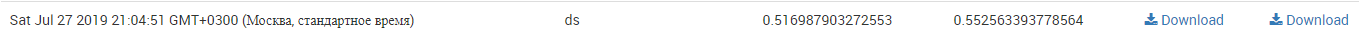# Data overview

I always start with EDA at the beginning of any project. So let’s have a look at the data. We have some unique id, the text of the review, the name of the drug and the sentiment (1 is negative, 2 is neutral, 0 is positive).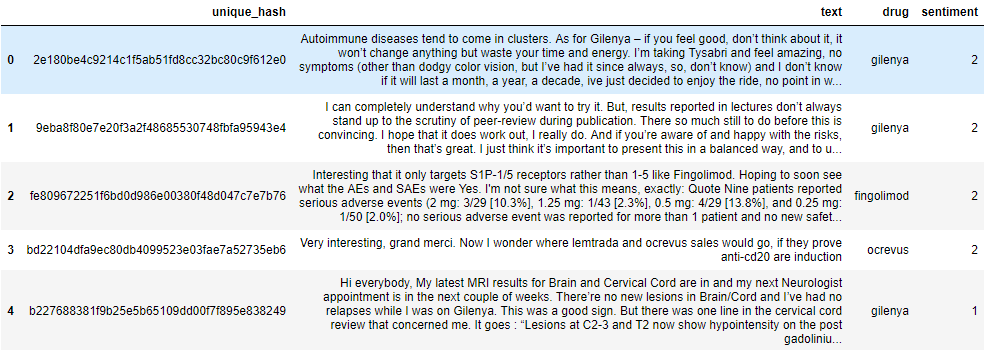People usually write about their illnesses, symptoms and drugs.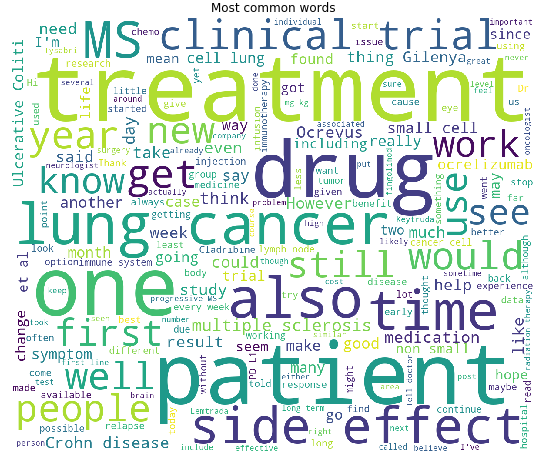Drugs in train and test dataset overlap, but not completely — some drugs are present in only one dataset.

As I wrote at the very beginning, most of the texts are short, but there are some huge texts, which could be here due to mistake.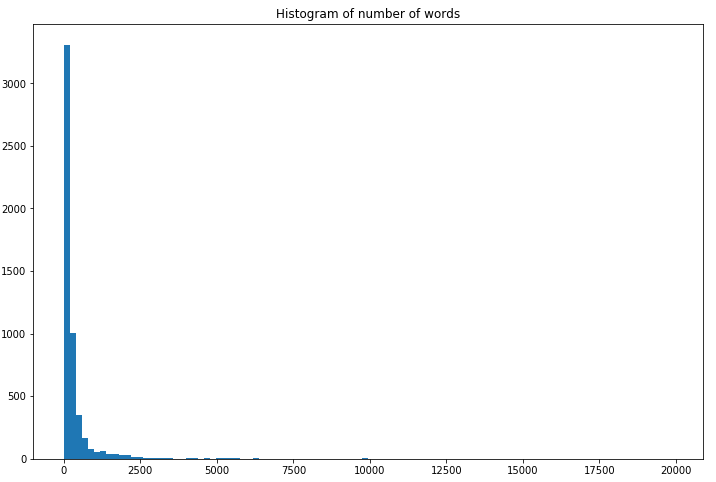# Setting up a baseline

It is usually a good idea to write a baseline which will be used later as a reference and to make sure that everything works correctly.

The main steps are the following:

• process data an prepare for modelling;
• build a model;
• perform validation (could be at the same time as training a model);

The basic way would be to skip any text preprocessing and use text vectorizer. I used TweetTokenizer from NLTK as it usually works better than default sklearn tokenizer. It can extract emoji and many other useful tokens. And it is almost always a good idea to use n-grams than single words.

We can directly use `sentiment` feature as target, as it is already numerical and starts with 0.

``````tokenizer = TweetTokenizer()
vectorizer = TfidfVectorizer(ngram_range=(1, 3), tokenizer=tokenizer.tokenize)
full_text = list(train['text'].values) + list(test['text'].values)
vectorizer.fit(full_text)
train_vectorized = vectorizer.transform(train['text'])
test_vectorized = vectorizer.transform(test['text'])
y = train['sentiment']
``````

We have a multiclass classification problem. There are two main approaches: building binary classifiers for each class vs other classes or binary classifiers for each pair of classes. I prefer the first approach and will use it with logistic regression.

``````logreg = LogisticRegression(class_weight='balanced')
ovr = OneVsRestClassifier(logreg)
``````

And now it is necessary to set up a way of checking the quality of our model. We could simply split train data, train model on one part and check quality on the other part. But I prefer using cross-validation. This way we train N models, validate N times and have a better measure of the model’s quality. So I use `cross_val_score` function with our model, use `f1_macro` metric, so that it will follow the leaderboard score and define simple 3 fold split.

``````scores = cross_val_score(ovr, train_vectorized, y, scoring='f1_macro', n_jobs=-1, cv=3)
print('Cross-validation mean f1_score {0:.2f}%, std {1:.2f}.'.format(np.mean(scores), np.std(scores)))
``````

The score on cross-validation is 0.4580. Now we can make prediction on test data, generate submission file and submit it.

``````pred = ovr.predict_proba(test_vectorized)
sub['sentiment'] = pred.argmax(1)
sub.to_csv('sub.csv', index=False)
``````

The result was 0.4499. This is a good beginning and shows that our validation scheme is good enough (has a similar score).

## Changing hyperparameters. First step.

Let’s try to improve the score. The first step is trying to change the preprocessing step. I used `TfidfVectorizer` with 1–3 ngrams and words as tokens. There are many possible ways to process text data:

• clean text. This could involve changing abbreviations to full words, removing numbers/punctuation/special symbols, fixing misspellings and so on;
• lemmatization or stemming to reduce the number of unique words;
• using letters/symbols as tokens (as opposed to using words);
• many other ideas;

As a first experiment, I changed `TfidfVectorizer`parameters to `TfidfVectorizer(ngram_range=(1, 5), analyzer='char'`. This increased my cross-validation score to 0.4849 and improved the score on public leaderboard up to 0.4624. Quite a good improvement for changing 1 line of code, eh?

Next idea: we can use both word and char tokens! We simply concatenate the matrices:

``````vectorizer = TfidfVectorizer(ngram_range=(1, 3), tokenizer=tokenizer.tokenize, stop_words='english')
full_text = list(train['text'].values) + list(test['text'].values)
vectorizer.fit(full_text)
train_vectorized = vectorizer.transform(train['text'])
test_vectorized = vectorizer.transform(test['text'])vectorizer1 = TfidfVectorizer(ngram_range=(1, 5), analyzer='char')
full_text = list(train['text'].values) + list(test['text'].values)
vectorizer1.fit(full_text)
train_vectorized1 = vectorizer1.transform(train['text'])
test_vectorized1 = vectorizer1.transform(test['text'])train_matrix = hstack((train_vectorized, train_vectorized1))
test_matrix = hstack((test_vectorized, test_vectorized1))
``````

This gave me 0.4930 cross-validation score and 0.4820 on leaderboard.

## Working with text

As I wrote at the beginning — the texts contain a lot of information and not all of it is useful. People could quote other texts, write long stories, compare several drugs and so on.

After several attempts I did the following:

``````train['new_text'] = train.apply(lambda row: ' '.join(\[i for i in row.text.lower().split('.') if row.drug in i]), axis=1)
``````

Now we have new texts, which contain only sentences where the relevant drug is mentioned. After this I tuned hyperparameters and ended with this vectorizer:

``````TfidfVectorizer(ngram_range=(1, 3),  max_df=0.75, min_df=10, sublinear_tf=True)
``````

This yielded 0.5206 score on cross-validation and 0.5279 on public leaderboard. It was my selected submit which gave me the 21th place on the leaderboard.

## Model interpreting

It is usually a good idea to see what and how model predicts, this could lead to some insights which could improve our models.

ELI5 can interpret our models and shows predictions in this way: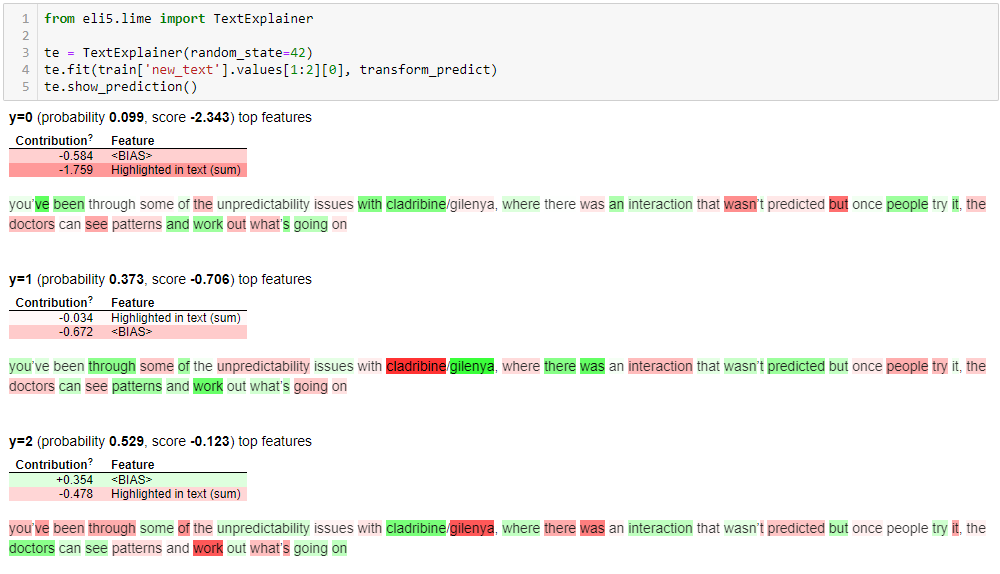Red words mean that this words decrease the probability of this class, green words increase the probability.

A recipe for a better solution

I have tried a lot of things to improve the score: different models (like SGD), hyperparameter optimization, text cleaning, undersampling, semi-supervised learning and other things. Let’s see how was my best solution created.

• Better text preprocessing

Cleaning text didn’t work for me, but I was able to improve my method of shortening the text. Now I use not only the sentence where the drug is mentioned, but also the next sentence — I think people will usually write something else after mentioning the drug for the first time. Also I take only first 10 sentences: most of the texts are within this limit, but there are some huge texts, which can make the training worse.

``````def get_text(row):
splitted_text = row.text.lower().split('.')
indices = [splitted_text.index(j) for j in [i for i in splitted_text if row.drug in i]]
full_indices = []
for i in indices:
full_indices.append(i)
if i < len(splitted_text) -1:
full_indices.append(i + 1)
full_indices = list(set(full_indices))
full_text = []
for i in full_indices:
full_text.append(splitted_text[i])
return ' '.join(full_text[-10:])
``````
• Hyperparameter optimization

Hyperparameter optimization is always important. Sklearn API allows to build pipelines and optimize them with a convenient syntax:

``````combined_features = FeatureUnion([('tfidf', TfidfVectorizer(ngram_range=(1, 3))),
('tfidf_char', TfidfVectorizer(ngram_range=(1, 3), analyzer='char'))])
pipeline = Pipeline([("features", combined_features),
('clf', OneVsRestClassifier(LogisticRegression(class_weight='balanced')))])parameters = {
'features__tfidf__max_df': (0.3, 0.75),
'features__tfidf_char__max_df': (0.3, 0.75),
'clf__estimator__C': (1.0, 10.0)
}
grid_search = GridSearchCV(pipeline, parameters, cv=folds,
n_jobs=-1, verbose=1, scoring='f1_macro')
grid_search.fit(train['new_text'], train['sentiment'])
``````

This way we can optimize parameters for two vectorizers and for a logistic regression model which is inside `OneVsRestClassifier`.

• Undersampling

I have decided that maybe class imbalance is too high and something should be done about it. There are many ways to deal with it: undersampling, oversampling, SMOTE, changing class disbalance. You can notice that I use logistic regression with balanced class weights, but it wasn’t enough. After several attempts I decided to do a simple undersampling and take random 2000 samples of neutral class.

• Semi-supervised learning

The idea is quite simple: after we train our model, it should give good predictions (well, at least we hope so :)), and most confident predictions should be correct, or at least most of them. I took 1000 top predictions and added them to train dataset. After training a new model on the increased dataset, I got 0.5169 on the public leaderboard. This submission was worth 0.5525 on private leaderboard, but I didn’t trust it enough to choose it.

# Conclusions

On the one hand, it is possible to get high results using more traditional ML approaches, on the other nowadays these approaches aren’t enough to beat deep learning with pretrained models. Does this mean there is no place for simpler models? I think that sometimes in business using logistic regression could be reasonable: it is faster to set up, easier to interpret and requires less resources. Nevertheless, in the long run using deep learning is a way to success.